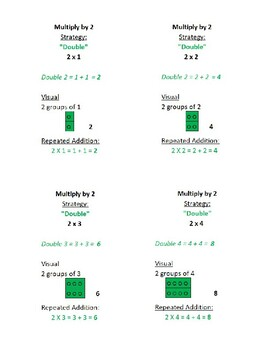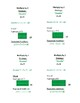# Multiplication Flashcards with Strategies and Visuals for 2 through 9Subject
Resource Type
File Type

PDF

(4 MB|44 pages)
Standards
• Product Description
• StandardsNEW

This product contains 64 quarter sheet flash cards of the multiples of 2 through 9 emphasizing strategies and visuals. There is only one flashcard and one example for multiplying by 1, 10, 11, and 12. There is also an extra flashcard about using the commutative property to help with multiplication.

As a math intervention teacher I find that many students either lack the ability to automatically retrieve multiplication facts and/or understand the strategies that can be used to multiply and/or the conceptual understanding of multiplication in general.

Apply properties of operations as strategies to multiply and divide. Examples: If 6 × 4 = 24 is known, then 4 × 6 = 24 is also known. (Commutative property of multiplication.) 3 × 5 × 2 can be found by 3 × 5 = 15, then 15 × 2 = 30, or by 5 × 2 = 10, then 3 × 10 = 30. (Associative property of multiplication.) Knowing that 8 × 5 = 40 and 8 × 2 = 16, one can find 8 × 7 as 8 × (5 + 2) = (8 × 5) + (8 × 2) = 40 + 16 = 56. (Distributive property.)
Interpret products of whole numbers, e.g., interpret 5 × 7 as the total number of objects in 5 groups of 7 objects each. For example, describe a context in which a total number of objects can be expressed as 5 × 7.
Total Pages
44 pages
N/A
Teaching Duration
1 month
Report this Resource to TpT
Reported resources will be reviewed by our team. Report this resource to let us know if this resource violates TpT’s content guidelines.